Teachoo Questions

Class 9
Chapter 8 Class 9 - Motion

## Find Displacement and Acceleration from Time 0-2 seconds, 2-10 secs, 10-12 seconds, 12-16 seconds. Also tell the type of motion in all the regions. And find the total displacement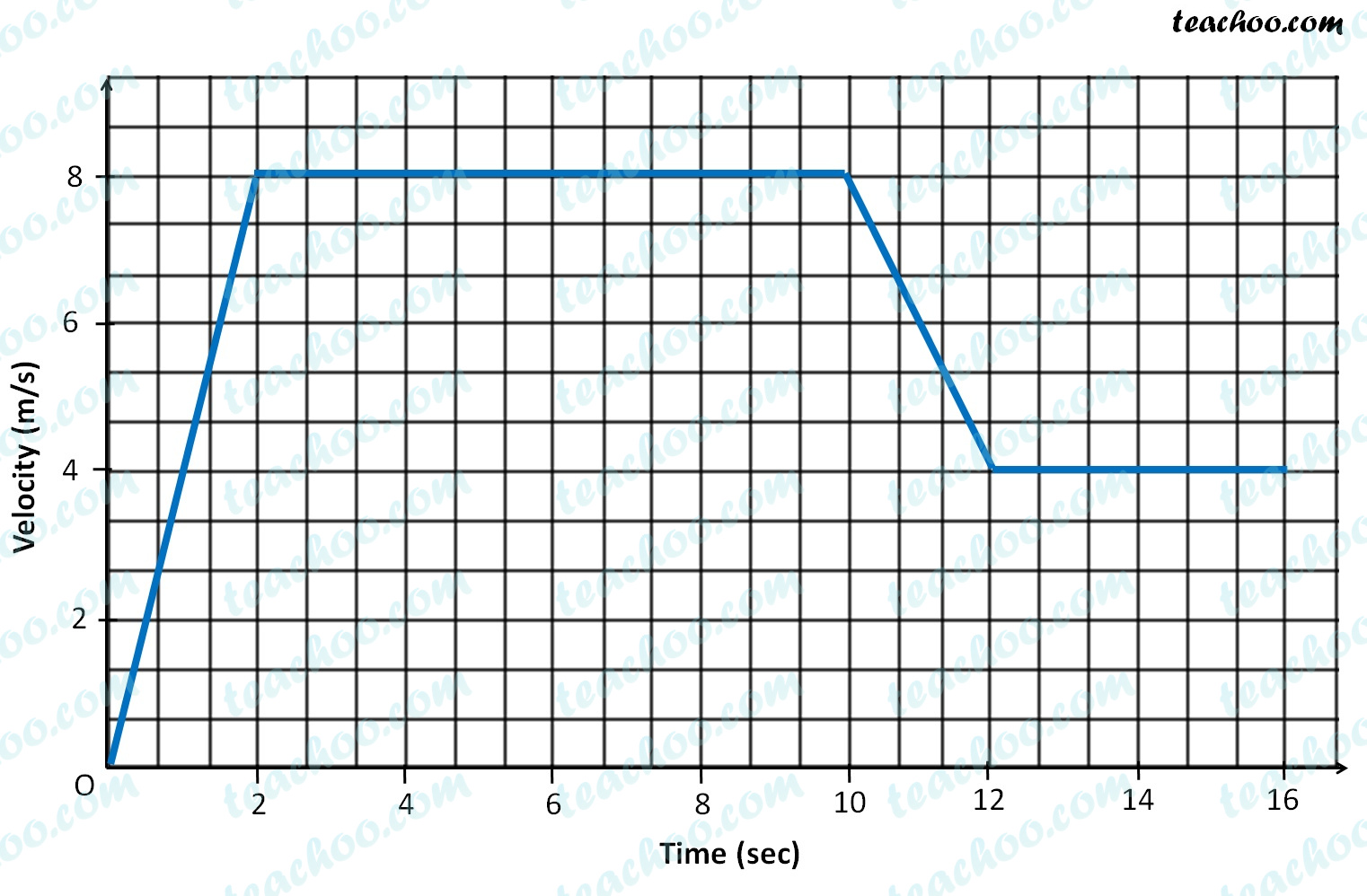Let’s mark the points and their coordinates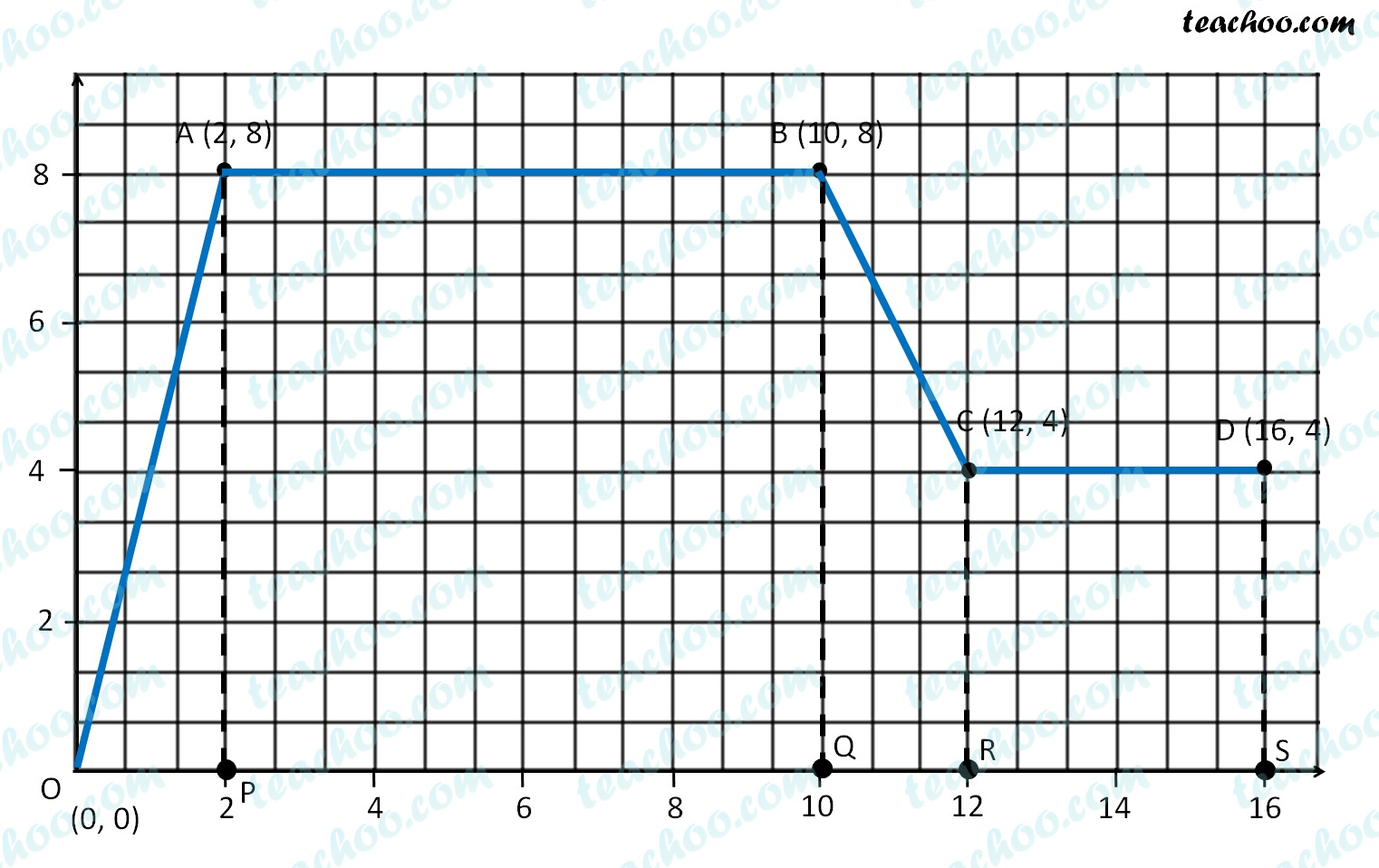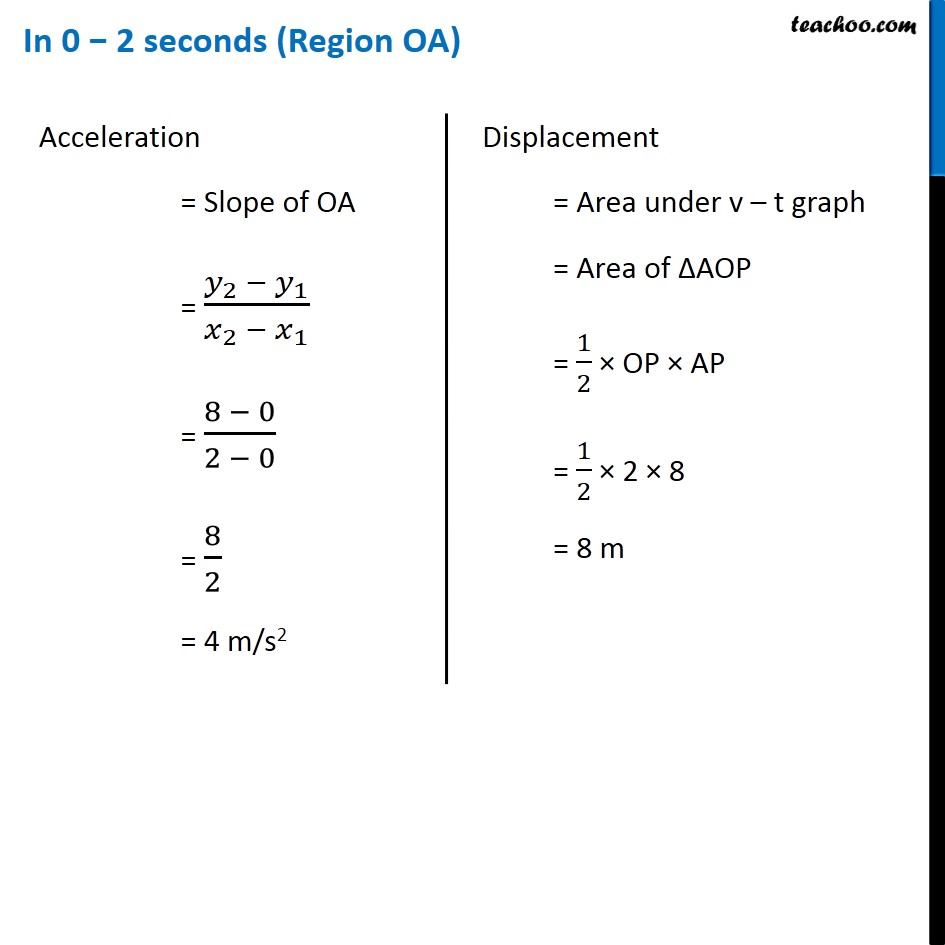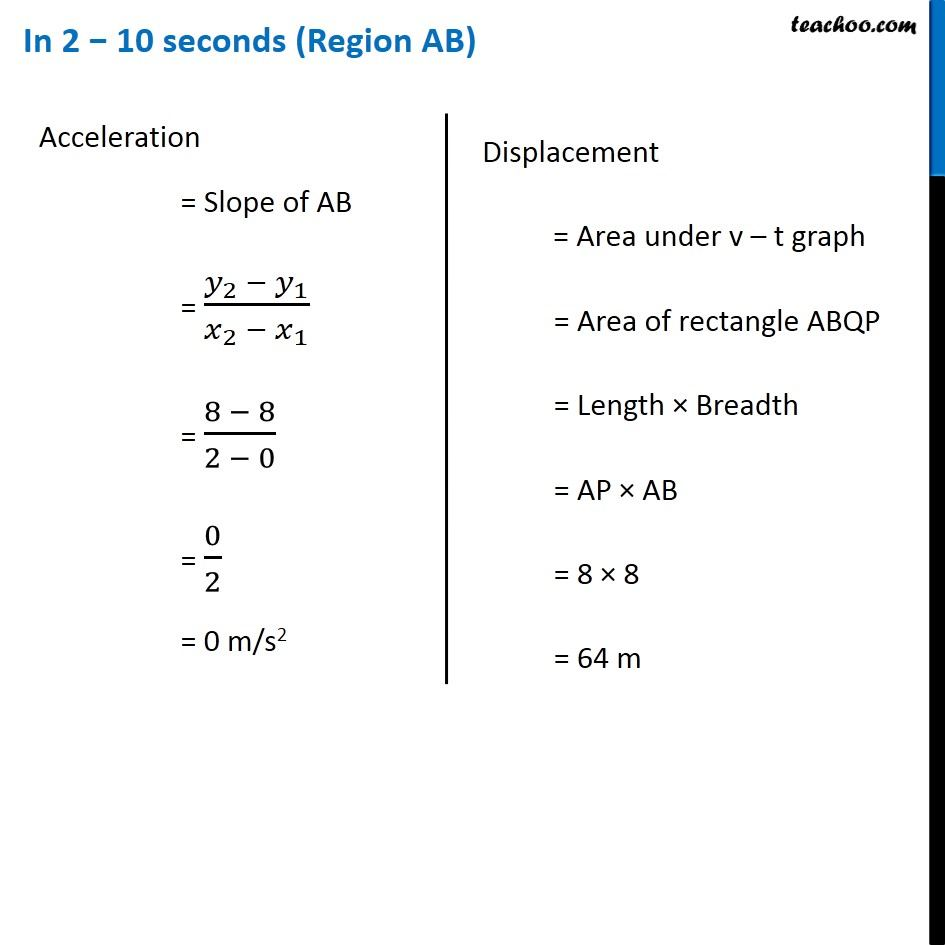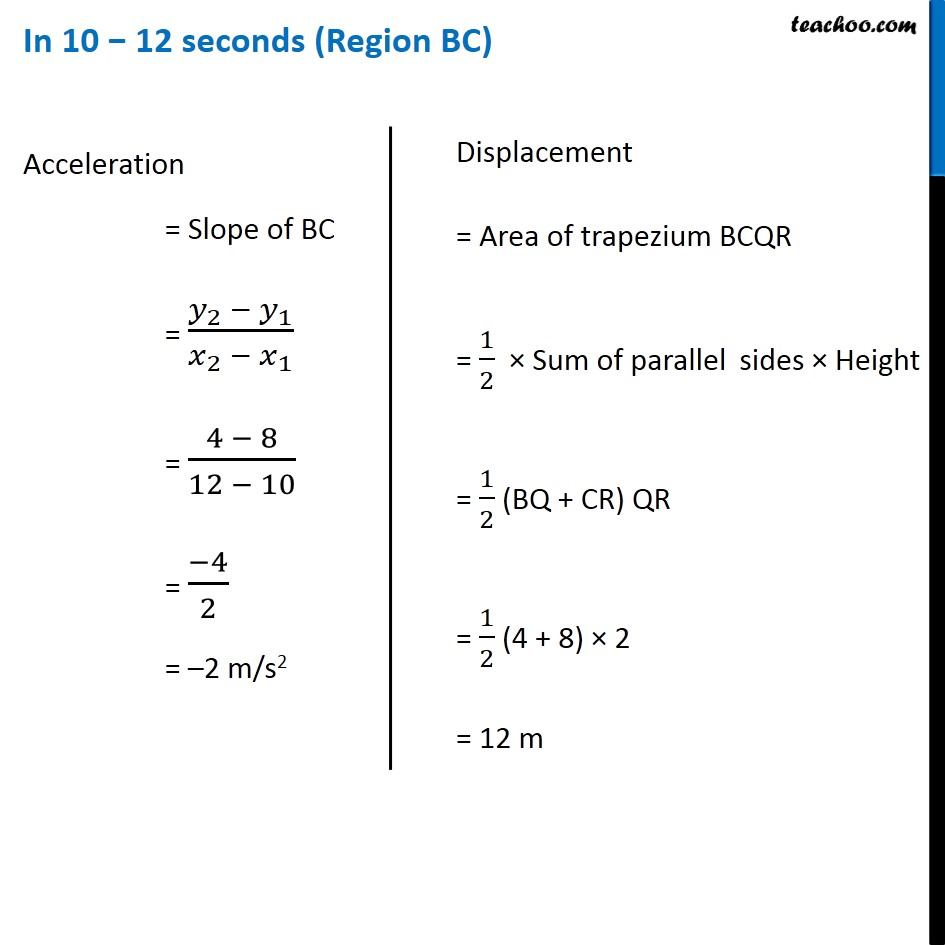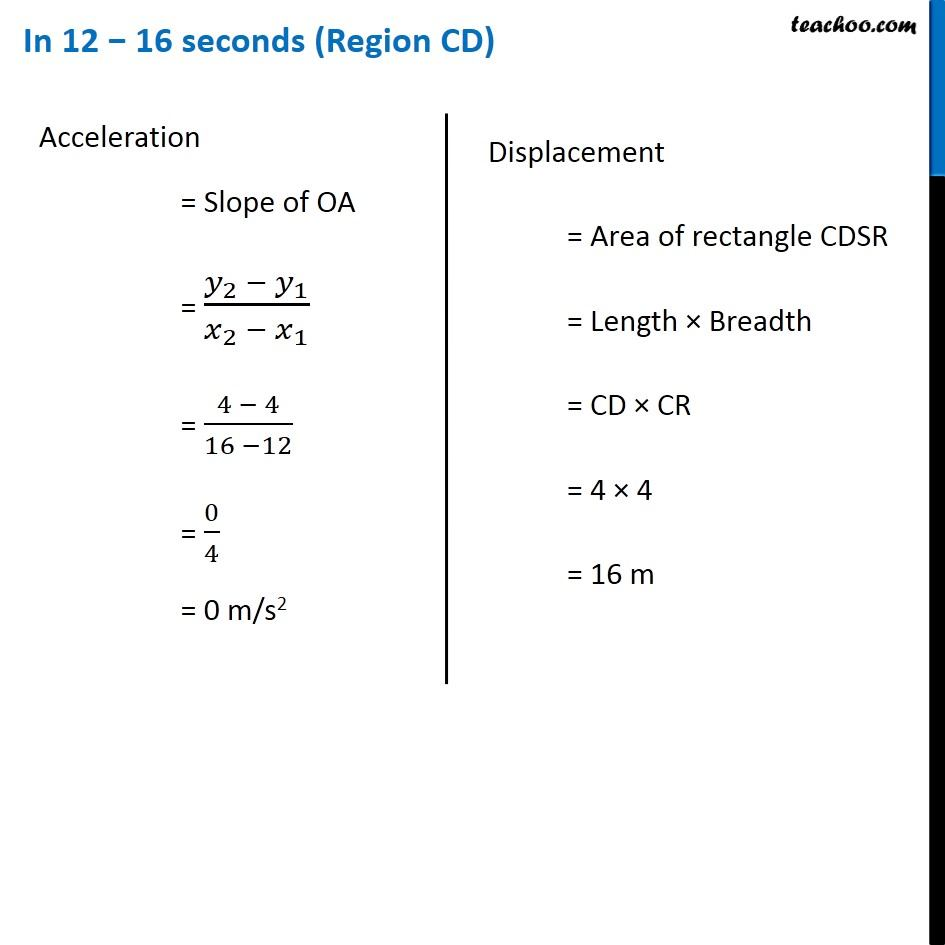Motion in region OA = Uniformly accelerated

Motion in region AB = Uniformly motion (No acceleration)

Motion in region BC = Uniformly retarded

Motion in region CD = Uniformly motion (No acceleration)

Total displacement

= Displacement in OA + Displacement in AB

+ Displacement in BC + Displacement in CD

= 8 + 64 + 12 + 16

= 100 m

Learn in your speed, with individual attention - Teachoo Maths 1-on-1 Class

### Transcript

In 2 − 10 seconds (Region AB) Acceleration = Slope of AB = (𝑦_2 − 𝑦_1)/(𝑥_2 − 𝑥_1 ) = (8 − 8)/(2 − 0) = 0/2 = 0 m/s2 Displacement = Area under v – t graph = Area of rectangle ABQP = Length × Breadth = AP × AB = 8 × 8 = 64 m In 10 − 12 seconds (Region BC) Acceleration = Slope of BC = (𝑦_2 − 𝑦_1)/(𝑥_2 − 𝑥_1 ) = (4 − 8)/(12 − 10) = (−4)/2 = –2 m/s2 Acceleration = Slope of BC = (𝑦_2 − 𝑦_1)/(𝑥_2 − 𝑥_1 ) = (4 − 8)/(12 − 10) = (−4)/2 = –2 m/s2 In 12 − 16 seconds (Region CD) Acceleration = Slope of OA = (𝑦_2 − 𝑦_1)/(𝑥_2 − 𝑥_1 ) = (4 − 4)/(16 −12) = 0/4 = 0 m/s2 Displacement = Area of rectangle CDSR = Length × Breadth = CD × CR = 4 × 4 = 16 m